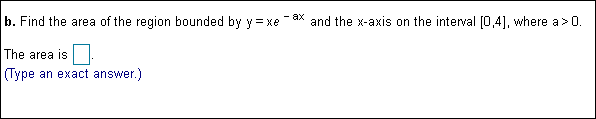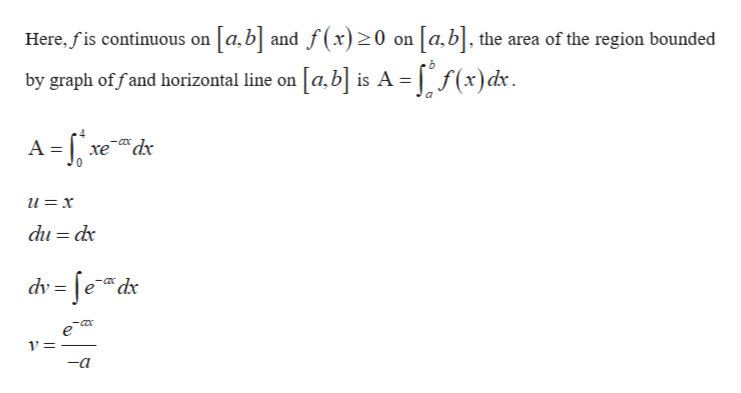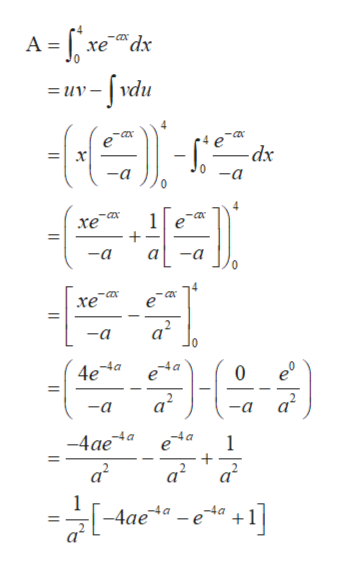b. Find the area of the region bounded by Y = xeax and the x-axis on the interval 0,41, where a> 0.The area is(Type an exact answer.)

Questionhelp_outlineImage Transcriptioncloseb. Find the area of the region bounded by Y = xe ax and the x-axis on the interval 0,41, where a> 0. The area is (Type an exact answer.) fullscreen
Step 1

Area of region which is bounded by f(x) and x-axis on [0,4] computed as follows.help_outlineImage TranscriptioncloseHere.fis continuous on [a,b] and f(x)20 on [a,b] the area of the region bounded by graph of fand horizontal line on [a,b] is A = [ /(x)dx A -xe xedr u=x du dx dv= fedx 1 = -a fullscreen
Step 2

Using integral by parts to s...help_outlineImage TranscriptioncloseA-ve w-/vdu -COx xedx =uv -dx 0a -a 4 -ax 1 хе -a -a -ax -ax хе 2. a -a 0 -4a 0 4ea e a2 -a -a -4a e -4ae a2 -4a + a2 a2 +1] e -4 a -4ae fullscreen

Want to see the full answer?

See Solution

Want to see this answer and more?

Our solutions are written by experts, many with advanced degrees, and available 24/7

See Solution
Tagged in

Integration